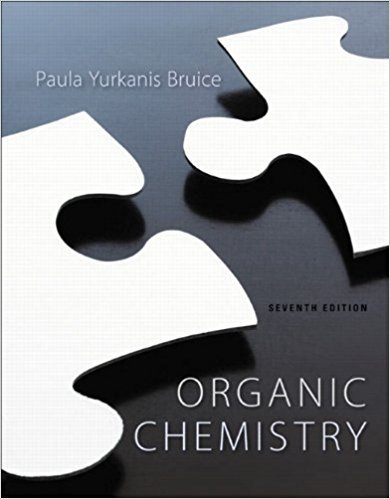×

# Does increasing the energy barrier for an SN2 reactionISBN: 9781269406772 80

## Solution for problem 4P Chapter 9

Organic Chemistry | 7th Edition

• Textbook Solutions
• 2901 Step-by-step solutions solved by professors and subject experts
• Get 24/7 help from StudySoup virtual teaching assistantsOrganic Chemistry | 7th Edition

4 5 0 386 Reviews
31
2
Problem 4P

Does increasing the energy barrier for an SN2 reaction increase or decrease the magnitude of the rate constant for the reaction?

Step-by-Step Solution:

Solution 4P

Step 1 of 1</p>Step 2 of 3

Step 3 of 3

##### ISBN: 9781269406772

This full solution covers the following key subjects: reaction, increase, decrease, Energy, constant. This expansive textbook survival guide covers 30 chapters, and 2818 solutions. Organic Chemistry was written by and is associated to the ISBN: 9781269406772. The answer to “Does increasing the energy barrier for an SN2 reaction increase or decrease the magnitude of the rate constant for the reaction?” is broken down into a number of easy to follow steps, and 21 words. Since the solution to 4P from 9 chapter was answered, more than 266 students have viewed the full step-by-step answer. The full step-by-step solution to problem: 4P from chapter: 9 was answered by , our top Chemistry solution expert on 09/04/17, 10:07PM. This textbook survival guide was created for the textbook: Organic Chemistry, edition: 7.

Unlock Textbook Solution

Does increasing the energy barrier for an SN2 reaction

×